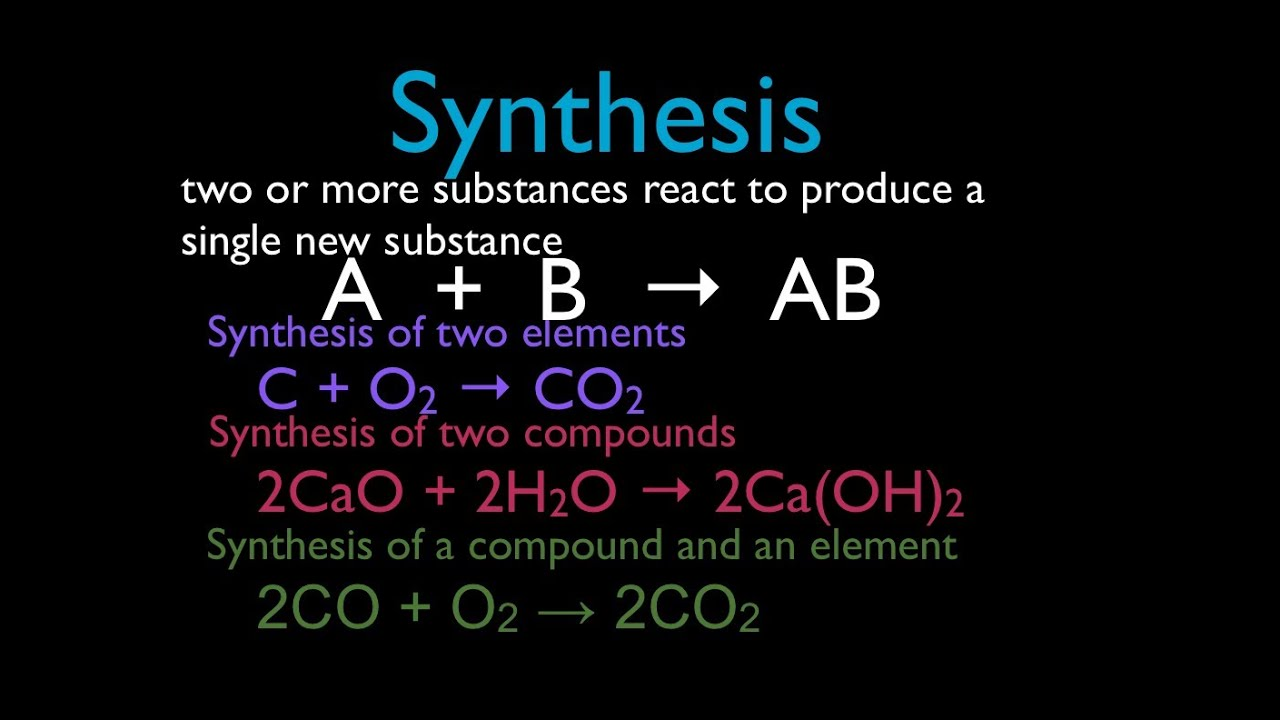# Aluminum can react with oxygen gas to produce aluminum oxide (Al_2O_3). What type of reaction is this?

Aug 16, 2017

This is a synthesis reaction.

#### Explanation:

We're given that aluminum ($\text{Al}$) can react with oxygen gas (${\text{O}}_{2}$; don't forget it's diatomic!) to produce aluminum oxide (${\text{Al"_2"O}}_{3}$).

The balanced chemical equation for this reaction is

ul(4"Al"(s) + 3"O"_2(g) rarr 2"Al"_2"O"_3(s)

A reaction in which two or more reactants combine to form only one product is called a synthesis reaction.

Here, there are two reactants ($\text{Al}$ and ${\text{O}}_{2}$) combining to form one product, ${\text{Al"_2"O}}_{3}$ (key word one), so this is a synthesis reaction.

Here is a useful image with more synthesis reaction examples: